# Class 10 NCERT Solutions- Chapter 9 Some Application of Trigonometry – Exercise 9.1 | Set 2

• Last Updated : 05 Apr, 2021

### Question 11. A TV tower stands vertically on a bank of a canal. From a point on the other bank directly opposite the tower, the angle of elevation of the top of the tower is 60°. From another point 20 m away from this point on the line joining this point to the foot of the tower, the angle of elevation of the top of the tower is 30° (see Fig.). Find the height of the tower and the width of the canal.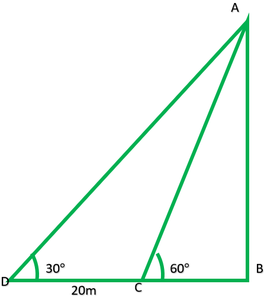Solution:

Attention reader! All those who say programming isn't for kids, just haven't met the right mentors yet. Join the  Demo Class for First Step to Coding Coursespecifically designed for students of class 8 to 12.

The students will get to learn more about the world of programming in these free classes which will definitely help them in making a wise career choice in the future.

In fig: AB = tower = ?

CB = canal = ?

In rt. ∆ABC,

tan60° =h/x = √3

h = √3 x          -(1)

In rt. ∆ABD= tan 30°= 1/√3

h = (x + 20)/√3          -(2)

From (1) and (2)

√3/1 = (x + 20)/√3

3x = x + 20

3x – x = 20

2x = 20

X = 20/2

X = 10

Width of the canal is 10m

Putting value of x in equation 1

h = √3 x

= 1.732(10)

= 17.32

Height of the tower 17.32m.

### Question 12. From the top of a 7 m high building, the angle of elevation of the top of a cable tower is 60° and the angle of depression of its foot is 45°. Determine the height of the tower.

Solution:

In fig: ED = building = 7m

AC = cable tower = ?

In rt ∆EDC,= tan45°

7/x = 1/1

DC = 7

Now, EB = DC = 7m

In rt. ∆ABE,= tan60°

AB/7 = √3/1

Height of tower = AC = AB + BC

7√3 + 7

= 7(√3 + 1)

= 7(1.732 + 1)

= 7(2.732)

Height of cable tower = 19.125m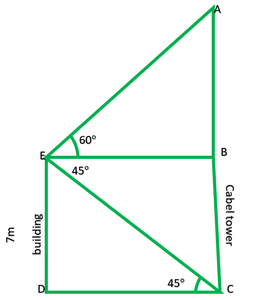### Question 13. As observed from the top of a 75 m high lighthouse from the sea-level, the angles of depression of two ships are 30° and 45°. If one ship is exactly behind the other on the same side of the lighthouse, find the distance between the two ships.

Solution:

In fig:

AB = lighthouse = 75m

D and C are two ships

DC = ?

In rt. ∆ABD,= tan30°

75/BD = 1/√3

BD = 75√3

In rt. ∆ABC= tan45°

75/BC = 1/1

BC = 75

DC = BD – BC

= 75√3 – 75

75(√3 – 1)

75(1.372 – 1)

34.900

Hence, distance between two sheep is 34.900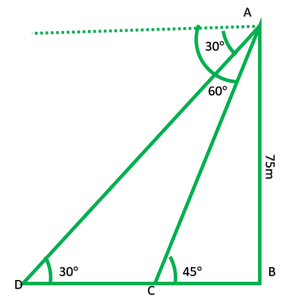### Question 14. A 1.2 m tall girl spots a balloon moving with the wind in a horizontal line at a height of 88.2 m from the ground. The height of 88.2 m from the ground. The angle of elevation of the balloon from the eyes of the girl at any instant is 60°. After some time, the angle of elevation reduces to 30° (see Fig.). Find the distance traveled by the balloon during the interval.

Solution:

In fig: AB = AC – BC

= 88.2 – 1.2

= 81m

In rt. ∆ABE= 87/EB = tan30°

87/EB = 1/√3

EB = 87√3

In rt. ∆FDE= tan60°

√3 ED = 87

ED = 87/√3

DB = DB – ED

87√3 – 87/√3

87(√3 – 1/√3)

= 87(3 – 1/√3)

= 87(2/√3) = 174/√3 * √3/√3

= 174 * √3/3 = 58√3

58 * 1.732 = 100.456m

Distance traveled by balloon is 100.456m

### Question 15. A straight highway leads to the foot of a tower. A man standing at the top of the tower observes a car at an angle of depression of 30°, which is approaching the foot of the tower with a uniform speed. Six seconds later, the angle of depression of the car is found to be 60°. Find the time taken by the car to reach the foot of the tower from this point.

Solution:

In fig: AB is tower

In rt. ∆ABD= tan30°= 1/√3

DB = √3 AB          -(1)

In rt. ∆ABC= tan60°

BC = AB/√3          -(2)

DC = DB – BC

= √3 AB – AB/√3

AB(3 – 1/√3)

CD = 2AB/√3S1 = S2

\frac{D1}{T1} = \frac{D2}{T2}2/√3AB/6 = AB/√3/t

2t = 6

t = 6/2

t = 3sec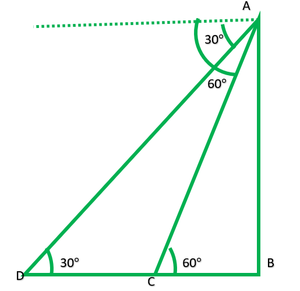### Question 16. The angles of elevation of the top of a tower from two points at a distance of 4 m and 9 m from the base of the tower and in the same straight line with it are complementary. Prove that the height of the tower is 6 m.

Solution:

In fig: AB is tower

To prove: AB = 6m

Given: BC = 4m          DB = 9m

In ∆ABC= tanθ

AB/4 = tanθ         -(1)

In ∆ABD= tan (90°-θ)

AB/9 = 1/ tanθ

9/AB = tanθ         -(2)

From (1) and (2)

AB/4 = 9/AB

AB2 = 36

AB = √36

AB = √(6 * 6)

AB = 6m

Height of the tower is 6m.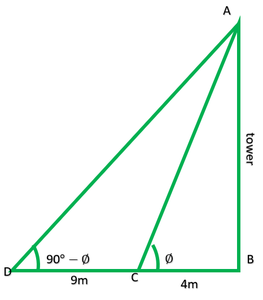My Personal Notes arrow_drop_up